# Quantum Harmonic Oscillator: Schrodinger Equation

The Schrodinger equation for a harmonic oscillator may be obtained by using the classical spring potential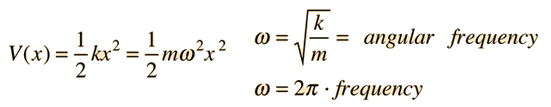The Schrodinger equation with this form of potential is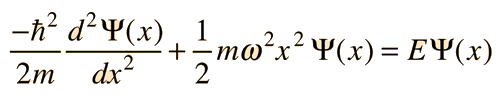Since the derivative of the wavefunction must give back the square of x plus a constant times the original function, the following form is suggested: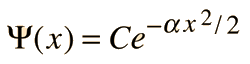Note that this form (a Gaussian function) satisfies the requirement of going to zero at infinity, making it possible to normalize the wavefunction.

Substituting this function into the Schrodinger equation and fitting the boundary conditions leads to the ground state energy for the quantum harmonic oscillator: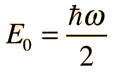Show

While this process shows that this energy satisfies the Schrodinger equation, it does not demonstrate that it is the lowest energy. One interesting way to show that is to demonstrate that that it is the lowest energy allowed by the uncertainty principle.

 The general solution to the Schrodinger equation leads to a sequence of evenly spaced energy levels characterized by a quantum number n.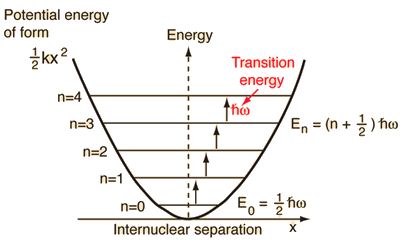The wavefunctions for the quantum harmonic oscillator contain the Gaussian form which allows them to satisfy the necessary boundary conditions at infinity. In the wavefunction associated with a given value of the quantum number n, the Gaussian is multiplied by a polynomial of order n called a Hermite polynomial. The expressions are simplified by making the substitution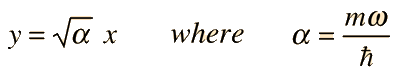The general formula for the normalized wavefunctions is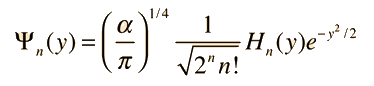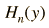= Hermite polynomial

The quantum harmonic oscillator is one of the foundation problems of quantum mechanics. It can be applied rather directly to the explanation of the vibration spectra of diatomic molecules, but has implications far beyond such simple systems. It is the foundation for the understanding of complex modes of vibration in larger molecules, the motion of atoms in a solid lattice, the theory of heat capacity, etc. In real systems, energy spacings are equal only for the lowest levels where the potential is a good approximation of the "mass on a spring" type harmonic potential. The anharmonic terms which appear in the potential for a diatomic molecule are useful for mapping the detailed potential of such systems.

Index

Schrodinger equation concepts

References
Beiser, Perspectives
Sec 8-7
Blatt
Sec 7-9

 HyperPhysics***** Quantum Physics R Nave
Go Back